# Intro to equilibrium abbrev alg

2 de Mar de 2011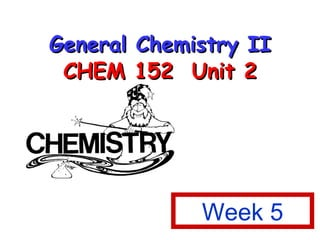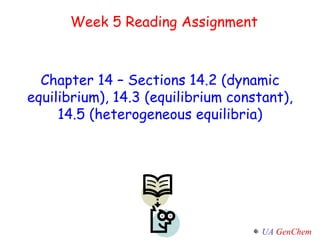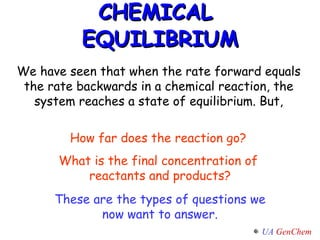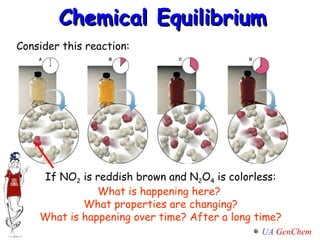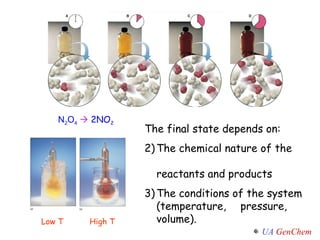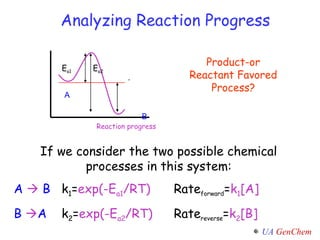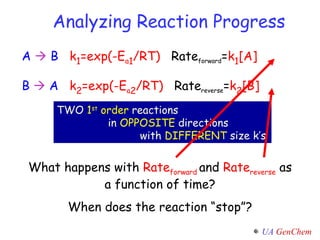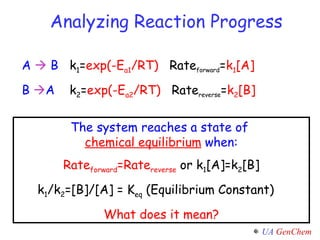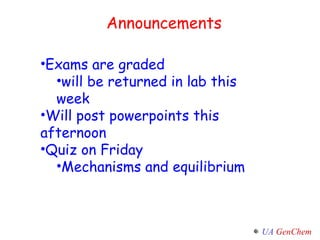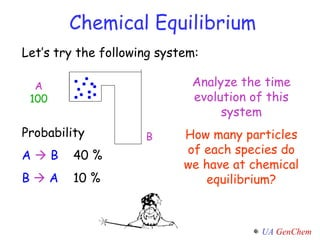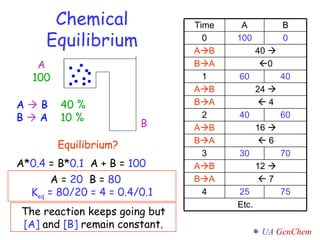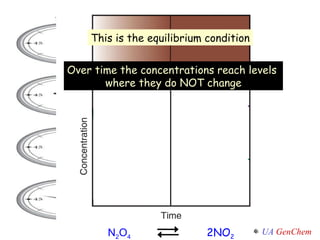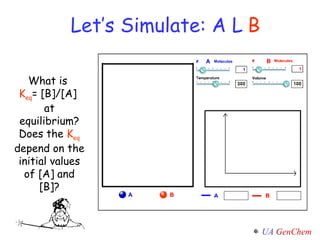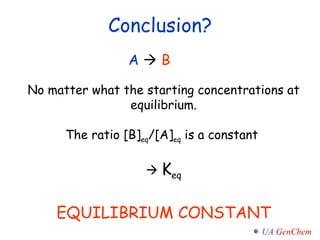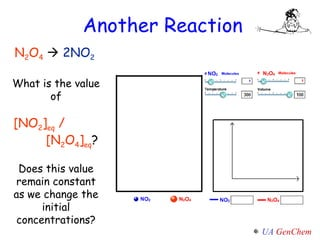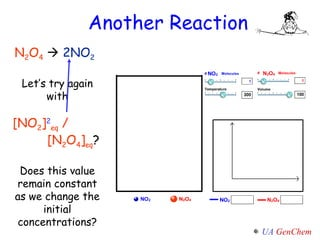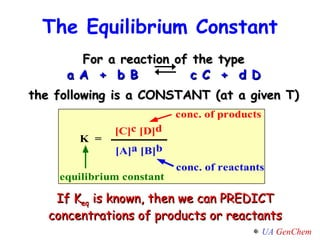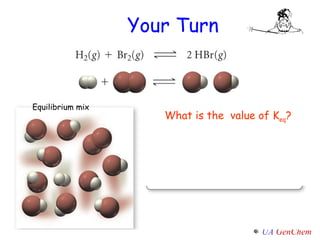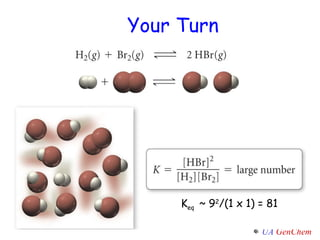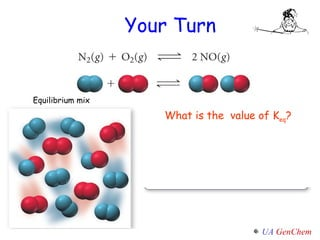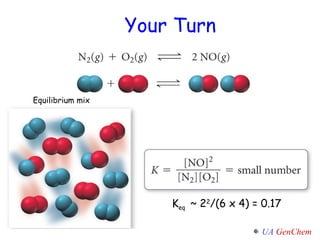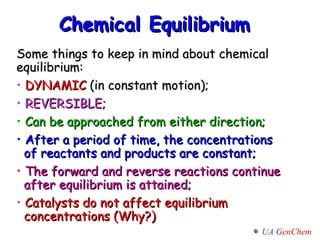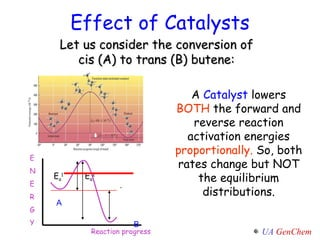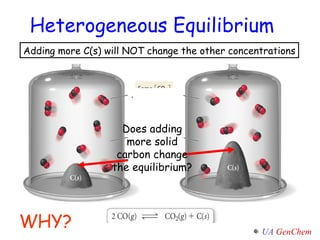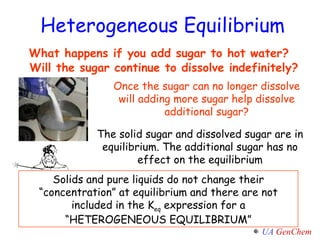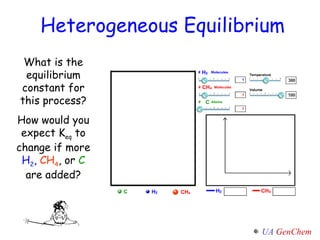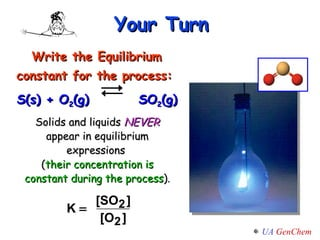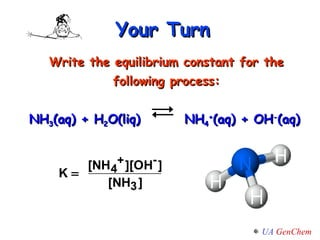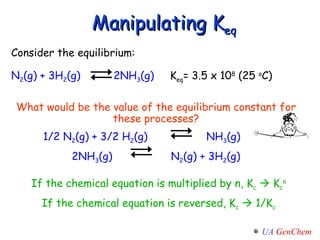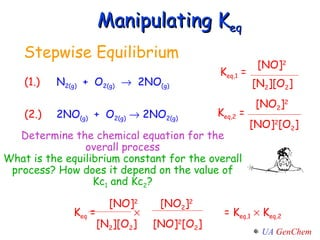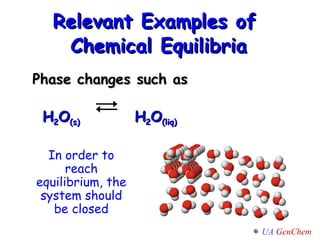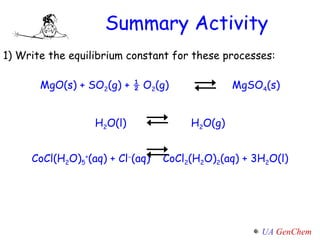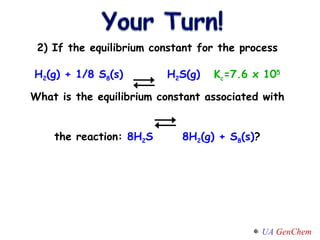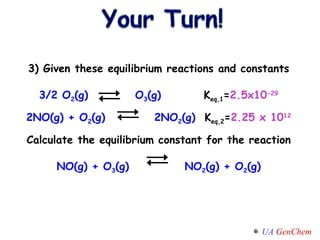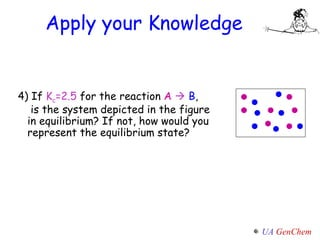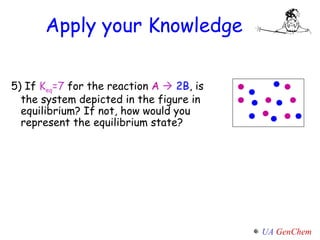1 de 40

### Intro to equilibrium abbrev alg

• 1. General Chemistry II CHEM 152 Unit 2 Week 5
• 2. Week 5 Reading Assignment Chapter 14 – Sections 14.2 (dynamic equilibrium), 14.3 (equilibrium constant), 14.5 (heterogeneous equilibria)
• 3. CHEMICAL EQUILIBRIUM How far does the reaction go? What is the final concentration of reactants and products? We have seen that when the rate forward equals the rate backwards in a chemical reaction, the system reaches a state of equilibrium. But, These are the types of questions we now want to answer.
• 4. Chemical Equilibrium Consider this reaction: If NO 2 is reddish brown and N 2 O 4 is colorless: What is happening here? What properties are changing? What is happening over time? After a long time?
• 5.
• 6. Analyzing Reaction Progress Product-or Reactant Favored Process? If we consider the two possible chemical processes in this system: A  B k 1 = exp(-E a1 /RT) Rate forward = k 1 [A] B  A k 2 = exp(-E a2 /RT) Rate reverse = k 2 [B] Reaction progress A B E a1 E a2 .
• 7. Analyzing Reaction Progress What happens with Rate forward and Rate reverse as a function of time? When does the reaction “stop”? A  B k 1 =exp(-E a 1 /RT) Rate forward = k 1 [A] B  A k 2 =exp(-E a 2 /RT) Rate reverse = k 2 [B] TWO 1 st order reactions in OPPOSITE directions with DIFFERENT size k’s
• 8. Analyzing Reaction Progress A  B k 1 = exp(-E a1 /RT) Rate forward = k 1 [A] B  A k 2 = exp(-E a2 /RT) Rate reverse = k 2 [B] The system reaches a state of chemical equilibrium when: Rate forward =Rate reverse or k 1 [A]=k 2 [B] k 1 /k 2 =[B]/[A] = K eq (Equilibrium Constant) What does it mean?
• 9. Week 5 Reading Assignment Chapter 14 – Sections 14.2 (dynamic equilibrium), 14.3 (equilibrium constant), 14.5 (heterogeneous equilibria)
• 10.
• 11. Chemical Equilibrium Let’s try the following system: A 100 B Probability A  B 40 % B  A 10 % Analyze the time evolution of this system How many particles of each species do we have at chemical equilibrium?
• 12. Chemical Equilibrium A 100 B A  B 40 % B  A 10 % Equilibrium? A* 0.4 = B* 0.1 A + B = 100 A = 20 B = 80 K eq = 80/20 = 4 = 0.4/0.1 The reaction keeps going but [A] and [B] remain constant. Time A B 0 100 0 A  B 40  B  A  0 1 60 40 A  B 24  B  A  4 2 40 60 A  B 16  B  A  6 3 30 70 A  B 12  B  A  7 4 25 75 Etc.
• 13. Over time the concentrations reach levels where they do NOT change This is the equilibrium condition N 2 O 4 2NO 2
• 14. Let’s Simulate: A  B What is K eq = [B]/[A] at equilibrium? Does the K eq depend on the initial values of [A] and [B]?
• 15. Conclusion? No matter what the starting concentrations at equilibrium. The ratio [B] eq /[A] eq is a constant  K eq EQUILIBRIUM CONSTANT A  B
• 16. Another Reaction N 2 O 4  2NO 2 What is the value of [NO 2 ] eq / [N 2 O 4 ] eq ? Does this value remain constant as we change the initial concentrations?
• 17. Another Reaction N 2 O 4  2NO 2 Let’s try again with [NO 2 ] 2 eq / [N 2 O 4 ] eq ? Does this value remain constant as we change the initial concentrations?
• 18.
• 19. Your Turn . Equilibrium mix What is the value of K eq ?
• 20. K eq ~ 9 2 /(1 x 1) = 81 Your Turn
• 21. Your Turn . . Equilibrium mix What is the value of K eq ?
• 22. Your Turn . Equilibrium mix K eq ~ 2 2 /(6 x 4) = 0.17
• 23.
• 24. Let us consider the conversion of cis (A) to trans (B) butene: Effect of Catalysts A Catalyst lowers BOTH the forward and reverse reaction activation energies proportionally. So, both rates change but NOT the equilibrium distributions. Reaction progress E N E R G Y A B E a 1 E a 2 .
• 25. Adding more C(s) will NOT change the other concentrations . Does adding more solid carbon change the equilibrium? Heterogeneous Equilibrium WHY?
• 26.
• 27. Heterogeneous Equilibrium What is the equilibrium constant for this process? How would you expect K eq to change if more H 2 , CH 4 , or C are added?
• 28.
• 29.
• 30.
• 31.
• 32. Manipulating K eq Consider the equilibrium: N 2 (g) + 3H 2 (g) 2NH 3 (g) K eq = 3.5 x 10 8 (25 o C) What would be the value of the equilibrium constant for these processes? 1/2 N 2 (g) + 3/2 H 2 (g) NH 3 (g) 2NH 3 (g) N 2 (g) + 3H 2 (g) If the chemical equation is multiplied by n, K c  K c n If the chemical equation is reversed, K c  1/K c
• 33. Stepwise Equilibrium (1.) N 2(g) + O 2(g)  2NO (g) (2.) 2NO (g) + O 2(g)  2NO 2(g) Manipulating K eq [NO] 2 K eq,1 = [N 2 ][O 2 ] [NO 2 ] 2 K eq,2 = [NO] 2 [O 2 ] [NO] 2 [NO 2 ] 2 K eq =  = K eq,1  K eq,2 [N 2 ][O 2 ] [NO] 2 [O 2 ] Determine the chemical equation for the overall process What is the equilibrium constant for the overall process? How does it depend on the value of Kc 1 and Kc 2 ?
• 34.
• 35.
• 36. Summary Activity 1) Write the equilibrium constant for these processes: MgO(s) + SO 2 (g) + ½ O 2 (g) MgSO 4 (s) H 2 O(l) H 2 O(g) CoCl(H 2 O) 5 + (aq) + Cl  (aq) CoCl 2 (H 2 O) 2 (aq) + 3H 2 O(l)
• 37. 2) If the equilibrium constant for the process H 2 (g) + 1/8 S 8 (s) H 2 S(g) K c =7.6 x 10 5 What is the equilibrium constant associated with the reaction: 8H 2 S 8H 2 (g) + S 8 (s) ?
• 38. 3) Given these equilibrium reactions and constants 3/2 O 2 (g) O 3 (g) K eq,1 = 2.5x10 -29 2NO(g) + O 2 (g) 2NO 2 (g) K eq,2 = 2.25 x 10 12 Calculate the equilibrium constant for the reaction NO(g) + O 3 (g) NO 2 (g) + O 2 (g)
• 39. Apply your Knowledge 4) If K c =2.5 for the reaction A  B , is the system depicted in the figure in equilibrium? If not, how would you represent the equilibrium state?
• 40. Apply your Knowledge 5) If K eq =7 for the reaction A  2B , is the system depicted in the figure in equilibrium? If not, how would you represent the equilibrium state?

### Notas del editor

1. Update for Tro.
2. Update for Tro.
3. Update for Tro.
4. Figure: 14-02 Title: Dynamic Equilibrium Caption: Equilibrium is reached in a chemical reaction when the concentrations of the reactants and products no longer change. The molecular images on the left depict the progress of the reaction H 2 ( g ) + I 2 ( g ) = 2 HI( g ). The graph on the right shows the concentrations of H 2 , I 2 , and HI as a function of time. When equilibrium is reached, both the forward and reverse reactions continue, but at equal rates, so the concentrations of the reactants and products remain constant.
5. Instructor: Use the simulation starting with different values for A and B. Have students calculate K=B/A to check that it is a constant. Turn the simulation off every time you want to change the number of particles to facilitate students’ understanding. If you leave the simulator on, the concentrations will adjust in real time.
6. Instructor: Use the simulation starting with different values for NO2 and N2O4. Have students calculate K=NO2/N2O4 to check if it is a constant. Turn the simulation off every time you want to change the number of particles to facilitate students’ understanding. If you leave the simulator on, the concentrations will adjust in real time.
7. Instructor: Use the simulation starting with different values for NO2 and N2O4. Have students calculate K=(NO2)(NO2)/N2O4 to check if it is a constant. Turn the simulation off every time you want to change the number of particles to facilitate students’ understanding. If you leave the simulator on, the concentrations will adjust in real time.
8. Tier 1
9. Tier 1
10. Talk to your neighbor – what questions do you have/ summarize your definition of equilibrium
11. Cut slide?
12. Tier 1
13. Talk to your neighbor – what questions do you have/ summarize your definition of equilibrium
14. Tier 1
15. Tier 1.5
16. Tier 1.5
17. Tier 2
18. Tier 2